CBSE Class 10 Sample Paper for 2022 Boards - Maths Standard [Term 2]

Class 10
Solutions of Sample Papers for Class 10 Boards

## Construct a pair of tangents to a circle of radius 4cm, which are inclined to each other at an angle of 60°.

This question is similar to Ex 11.2,4 - Chapter 11 Class 10 - Constructions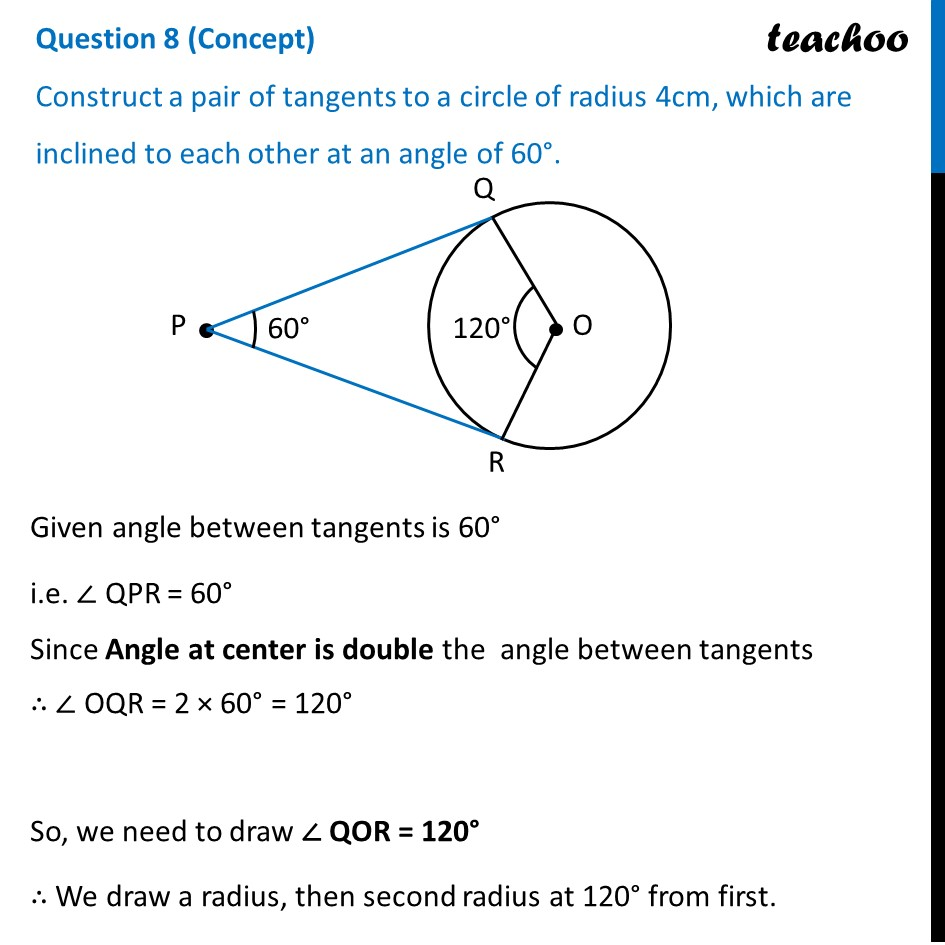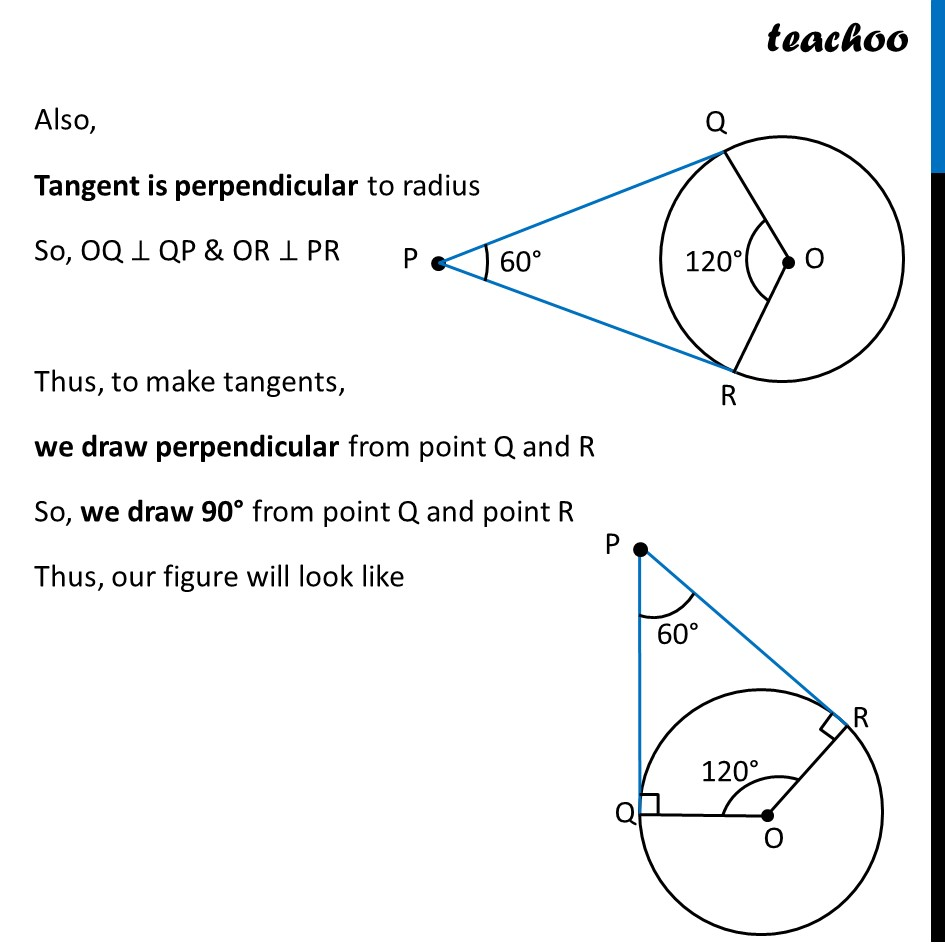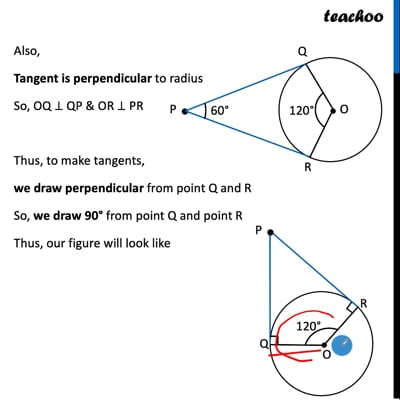This video is only available for Teachoo black users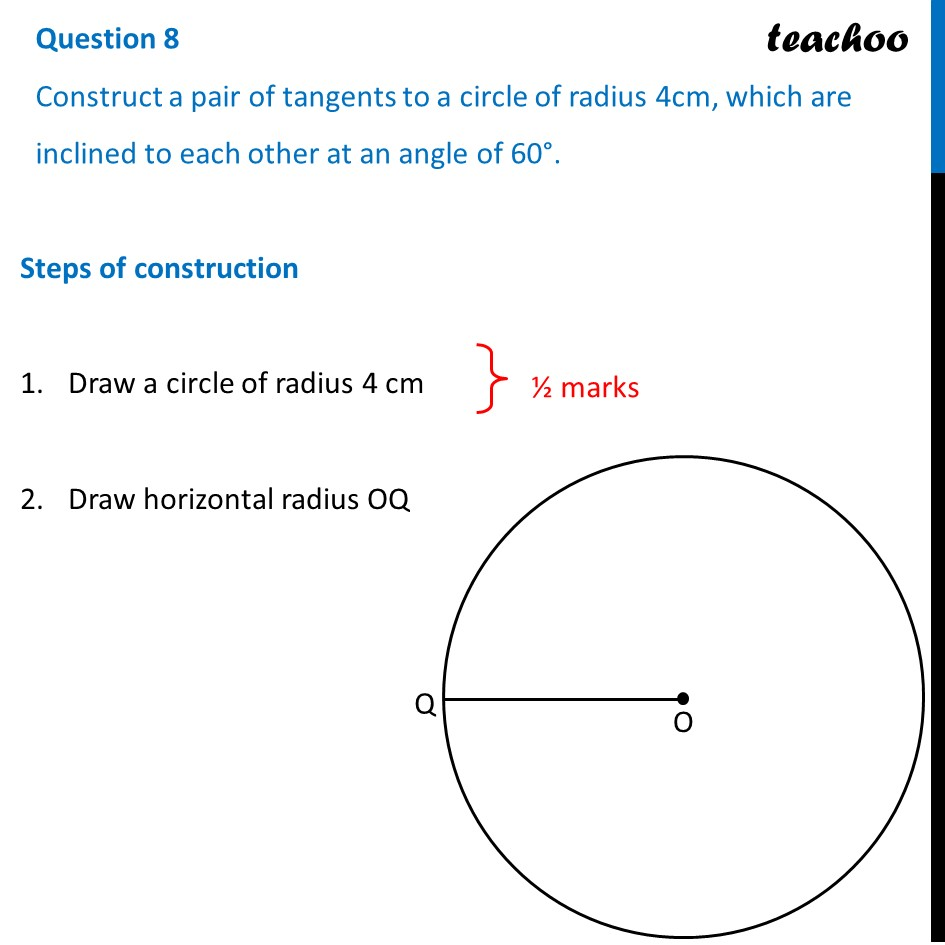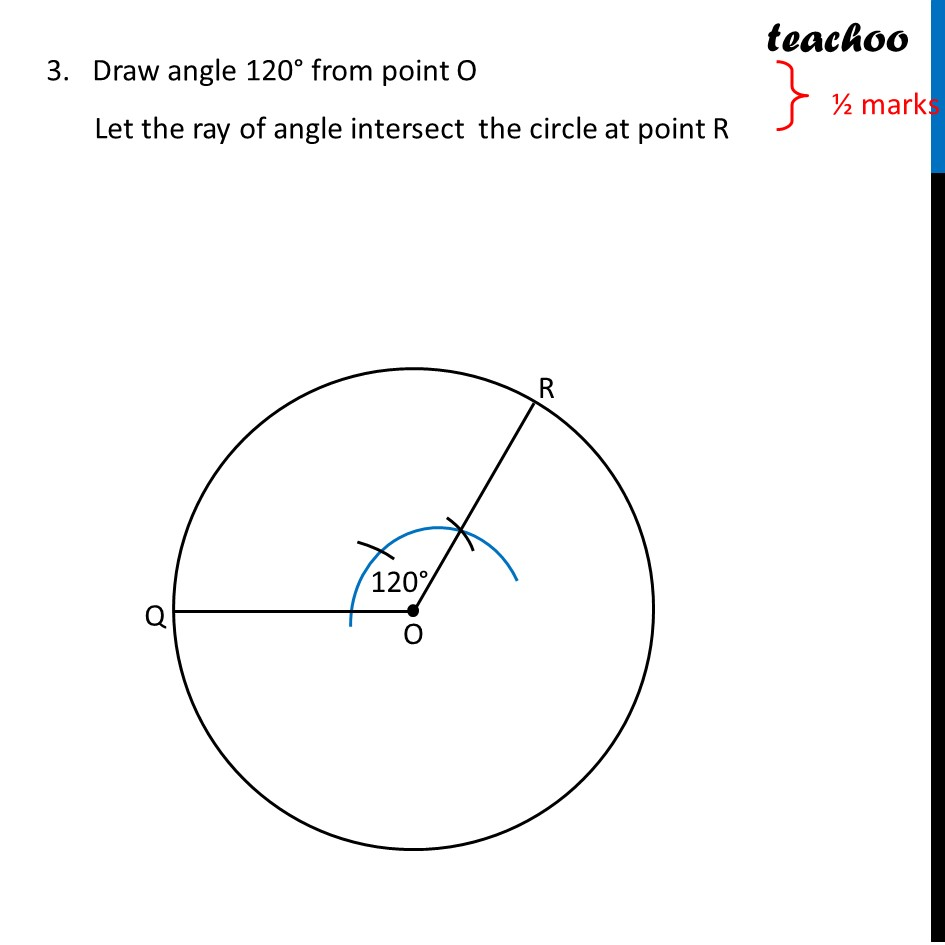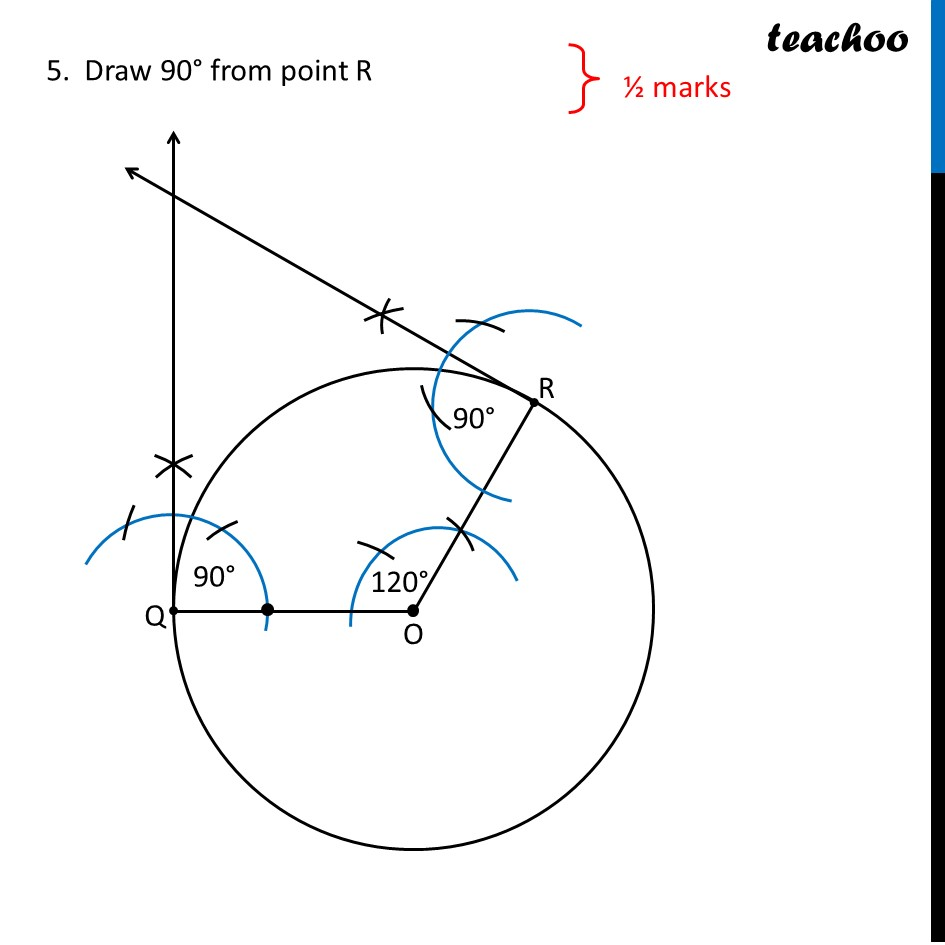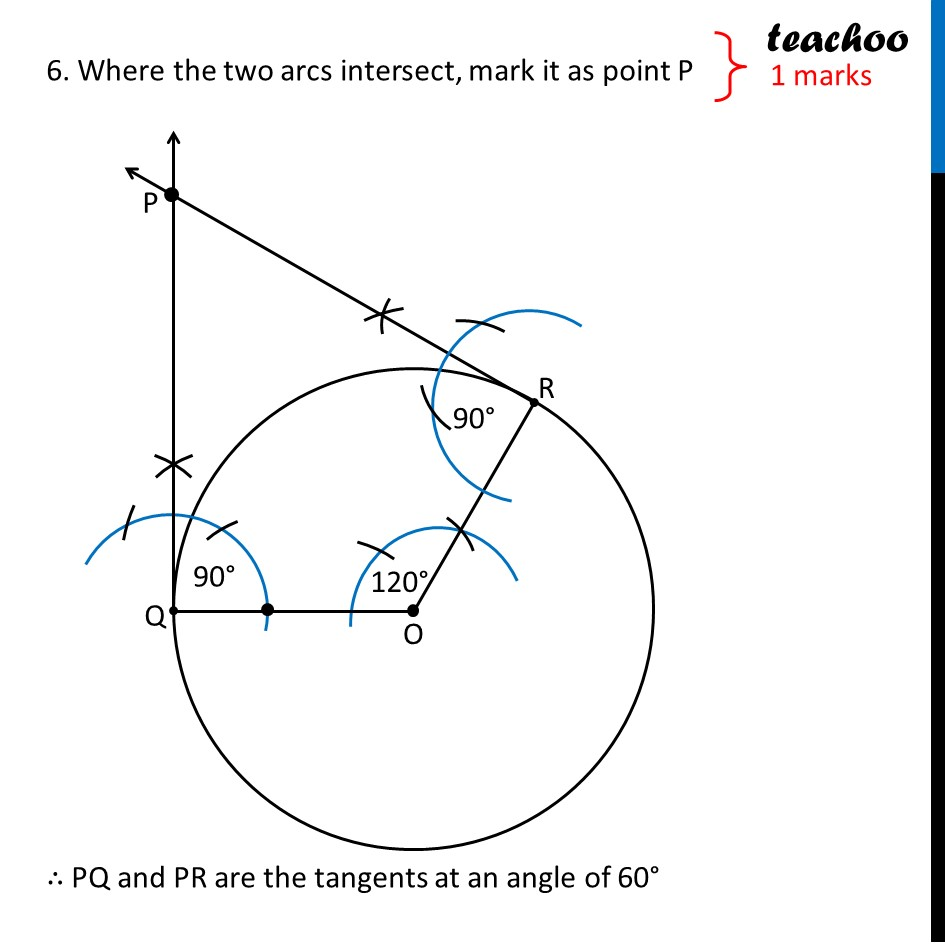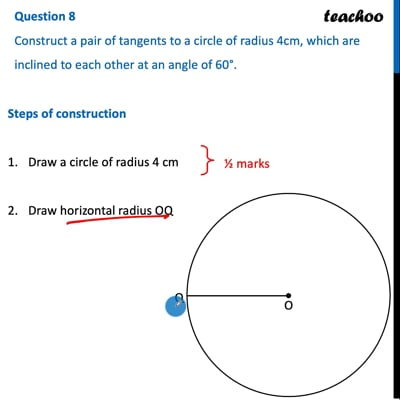This video is only available for Teachoo black users

### Transcript

Construct a pair of tangents to a circle of radius 4cm, which are inclined to each other at an angle of 60°. Given angle between tangents is 60° i.e. ∠ QPR = 60° Since Angle at center is double the angle between tangents ∴ ∠ OQR = 2 × 60° = 120° So, we need to draw ∠ QOR = 120° ∴ We draw a radius, then second radius at 120° from first. Also, Tangent is perpendicular to radius So, OQ ⊥ QP & OR ⊥ PR Thus, to make tangents, we draw perpendicular from point Q and R So, we draw 90° from point Q and point R Thus, our figure will look like Question 8 Construct a pair of tangents to a circle of radius 4cm, which are inclined to each other at an angle of 60°. Steps of construction Draw a circle of radius 4 cm Draw horizontal radius OQ 3. Draw angle 120° from point O Let the ray of angle intersect the circle at point R Now, draw 90° from point Q 5. Draw 90° from point R 6. Where the two arcs intersect, mark it as point P ∴ PQ and PR are the tangents at an angle of 60°